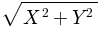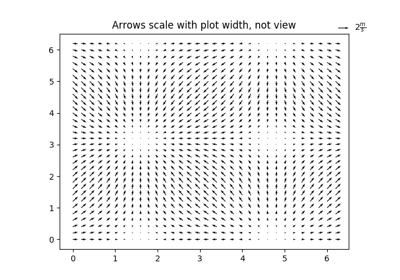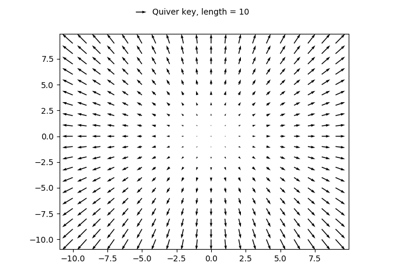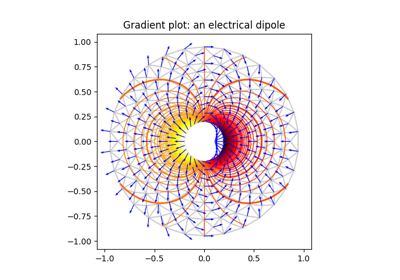# matplotlib.axes.Axes.quiver¶

`Axes.``quiver`(*args, data=None, **kw)[source]

Plot a 2-D field of arrows.

Call signatures:

```quiver(U, V, **kw)
quiver(U, V, C, **kw)
quiver(X, Y, U, V, **kw)
quiver(X, Y, U, V, C, **kw)
```

U and V are the arrow data, X and Y set the location of the arrows, and C sets the color of the arrows. These arguments may be 1-D or 2-D arrays or sequences.

If X and Y are absent, they will be generated as a uniform grid. If U and V are 2-D arrays and X and Y are 1-D, and if `len(X)` and `len(Y)` match the column and row dimensions of U, then X and Y will be expanded with `numpy.meshgrid()`.

The default settings auto-scales the length of the arrows to a reasonable size. To change this behavior see the scale and scale_units kwargs.

The defaults give a slightly swept-back arrow; to make the head a triangle, make headaxislength the same as headlength. To make the arrow more pointed, reduce headwidth or increase headlength and headaxislength. To make the head smaller relative to the shaft, scale down all the head parameters. You will probably do best to leave minshaft alone.

linewidths and edgecolors can be used to customize the arrow outlines.

Parameters: X : 1D or 2D array, sequence, optional The x coordinates of the arrow locations Y : 1D or 2D array, sequence, optional The y coordinates of the arrow locations U : 1D or 2D array or masked array, sequence The x components of the arrow vectors V : 1D or 2D array or masked array, sequence The y components of the arrow vectors C : 1D or 2D array, sequence, optional The arrow colors units : [ 'width' | 'height' | 'dots' | 'inches' | 'x' | 'y' | 'xy' ] The arrow dimensions (except for length) are measured in multiples of this unit. 'width' or 'height': the width or height of the axis 'dots' or 'inches': pixels or inches, based on the figure dpi 'x', 'y', or 'xy': respectively X, Y, orin data units The arrows scale differently depending on the units. For 'x' or 'y', the arrows get larger as one zooms in; for other units, the arrow size is independent of the zoom state. For 'width or 'height', the arrow size increases with the width and height of the axes, respectively, when the window is resized; for 'dots' or 'inches', resizing does not change the arrows. angles : [ 'uv' | 'xy' ], array, optional Method for determining the angle of the arrows. Default is 'uv'. 'uv': the arrow axis aspect ratio is 1 so that if U*==*V the orientation of the arrow on the plot is 45 degrees counter-clockwise from the horizontal axis (positive to the right). 'xy': arrows point from (x,y) to (x+u, y+v). Use this for plotting a gradient field, for example. Alternatively, arbitrary angles may be specified as an array of values in degrees, counter-clockwise from the horizontal axis. Note: inverting a data axis will correspondingly invert the arrows only with `angles='xy'`. scale : None, float, optional Number of data units per arrow length unit, e.g., m/s per plot width; a smaller scale parameter makes the arrow longer. Default is None. If None, a simple autoscaling algorithm is used, based on the average vector length and the number of vectors. The arrow length unit is given by the scale_units parameter scale_units : [ 'width' | 'height' | 'dots' | 'inches' | 'x' | 'y' | 'xy' ], None, optional If the scale kwarg is None, the arrow length unit. Default is None. e.g. scale_units is 'inches', scale is 2.0, and `(u,v) = (1,0)`, then the vector will be 0.5 inches long. If scale_units is 'width'/'height', then the vector will be half the width/height of the axes. If scale_units is 'x' then the vector will be 0.5 x-axis units. To plot vectors in the x-y plane, with u and v having the same units as x and y, use `angles='xy', scale_units='xy', scale=1`. width : scalar, optional Shaft width in arrow units; default depends on choice of units, above, and number of vectors; a typical starting value is about 0.005 times the width of the plot. headwidth : scalar, optional Head width as multiple of shaft width, default is 3 headlength : scalar, optional Head length as multiple of shaft width, default is 5 headaxislength : scalar, optional Head length at shaft intersection, default is 4.5 minshaft : scalar, optional Length below which arrow scales, in units of head length. Do not set this to less than 1, or small arrows will look terrible! Default is 1 minlength : scalar, optional Minimum length as a multiple of shaft width; if an arrow length is less than this, plot a dot (hexagon) of this diameter instead. Default is 1. pivot : [ 'tail' | 'mid' | 'middle' | 'tip' ], optional The part of the arrow that is at the grid point; the arrow rotates about this point, hence the name pivot. color : [ color | color sequence ], optional This is a synonym for the `PolyCollection` facecolor kwarg. If C has been set, color has no effect.

`quiverkey`
Add a key to a quiver plot

Notes

Additional `PolyCollection` keyword arguments:

Property Description
`agg_filter` a filter function, which takes a (m, n, 3) float array and a dpi value, and returns a (m, n, 3) array
`alpha` float or None
`animated` bool
`antialiased` bool or sequence of bools
`array` ndarray
`capstyle` {'butt', 'round', 'projecting'}
`clim` a length 2 sequence of floats; may be overridden in methods that have `vmin` and `vmax` kwargs.
`clip_box` `Bbox`
`clip_on` bool
`clip_path` [(`Path`, `Transform`) | `Patch` | None]
`cmap` colormap or registered colormap name
`color` matplotlib color arg or sequence of rgba tuples
`contains` callable
`edgecolor` color or sequence of colors
`facecolor` color or sequence of colors
`figure` `Figure`
`gid` str
`hatch` {'/', '\', '|', '-', '+', 'x', 'o', 'O', '.', '*'}
`in_layout` bool
`joinstyle` {'miter', 'round', 'bevel'}
`label` object
`linestyle` {'-', '--', '-.', ':', '', (offset, on-off-seq), ...}
`linewidth` float or sequence of floats
`norm` `Normalize`
`offset_position` {'screen', 'data'}
`offsets` float or sequence of floats
`path_effects` `AbstractPathEffect`
`picker` None or bool or float or callable
`pickradius` unknown
`rasterized` bool or None
`sketch_params` (scale: float, length: float, randomness: float)
`snap` bool or None
`transform` `Transform`
`url` str
`urls` List[str] or None
`visible` bool
`zorder` float

## Examples using `matplotlib.axes.Axes.quiver`¶Demonstration of advanced quiver and quiverkey functionsQuiver Simple Demo